• 唯一百姓论文网官方网站（其他网站均属假冒）。工作时间9：00-17:00，周末休息，QQ：1647439496。电话联系：15389037508(同微信)

# 基于集总参数法的振动台基础设计

、、、、、等系数可查询我国现行《动力机器基础设计规范》（以下简称“动规”）获得。由于液压振动台基础位于地基承载力为250KN/㎡的漂石层查表可得=49000KN/㎡，=0.7=34300 KN/㎡，=2.15=105350 KN/㎡。又=A、=A、=，为基础底面通过形心轴的惯性矩现需确定A和值。

（1）
（2）
（3）

1、输入式（2）与式（3），代码如下：
ODE:=866.365 diff(f(t),t\$2)+34300 diff(f(t),t)-98372 diff(g(t),t)+2195200 f(t)-6295833.6 g(t)=258 sin(t),1781555 diff(g(t),t)+105350 diff(f(t),t)+ 35924350 g(t)-6295833.9 f(t)=805 sin(t)
2、输入边界条件，由于液压振动台由静止开始启动，因此初始位移和速度均为0有：
Initvals:=f(0)=0,g(0)=0,(0)=0,(0)=0
3、求解，由于该方程组的解析解相当复杂且最终需要的为实际位移的数值，因此直接求数值解，有：
funcs:={f(t),g(t)}
F:=dsolve({ODE,initvals},funcs,type=numer)
4、结果输出，采用plots[odeplot]函数输出随时间t变化的函数图像。
P1=plots[odeplot](F,[t,f(t)],1..40);p2= plots[odeplot](F,[t,g(t)],1..40)

## 期刊巡展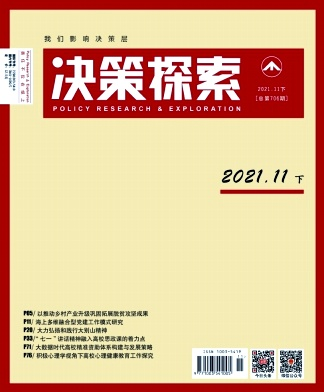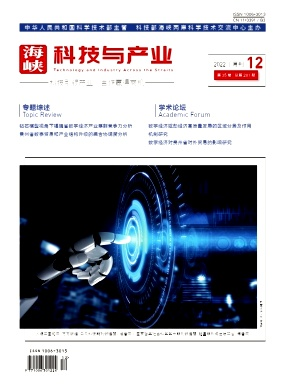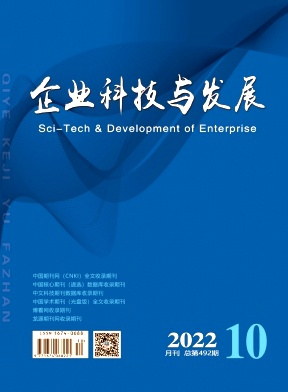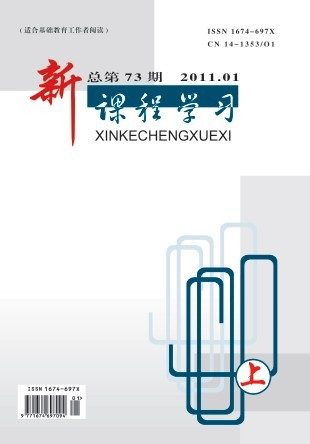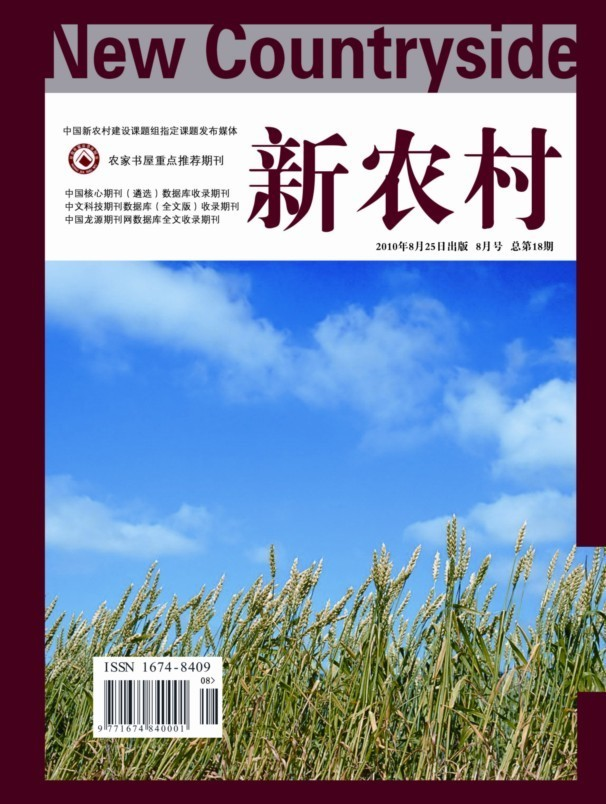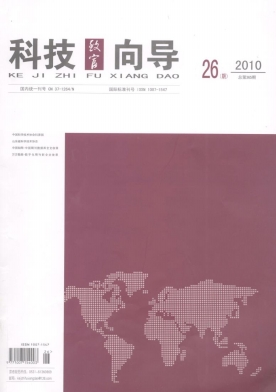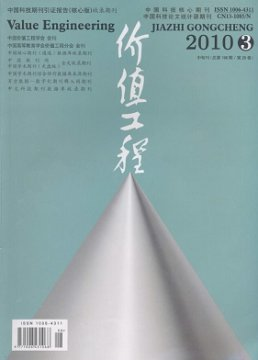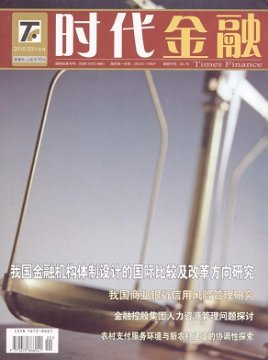《华夏教师》 《全国流通经 《才智》征稿 《决策探索》 《海峡科技与 《企业科技与 中学生数理化 新课程学习 《新农村》杂 《科技致富向 《价值工程》 《科技创新导 《时代金融》 《大众商务》